This section is Section 2 of 3.

Speed as well as accuracy is important in this section. Work quickly, or you might not finish the paper. There are no  penalties for incorrect responses, only marks for correct answers, so you should attempt all 27 questions. Each question is worth one mark.

You must complete the answers within the time limit. Calculators are NOT permitted.

Good Luck!

Note – if press “End Exam” you can access full worked solutions for all past paper questions

The table shows the percentages of some substances measured in fluid taken from a human kidney.

 substance % water 99 proteins 0 glucose 0.1 urea 0.03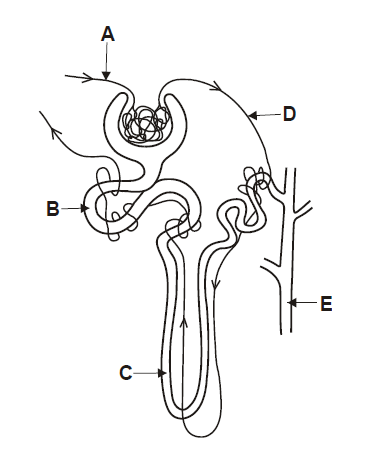1. Which letter on the diagram shows the most likely source of the fluid?
0
0

## Explanation

Nearly all of glucose is reabsorbed in the proximal convoluted tubule(Option B) so the fluid must have been from here.

It cannot be Option B or D as fluid flowing through those structures are blood which are abundant in protein.

Post Comment

Element x is in the second period of the Periodic Table. An atom of element y has six more protons than an atom of element x.

2. Which statement must be correct?
0
0

## Explanation

Post Comment

A detector of radioactivity shows background radiation to be 20 counts per minute with no radioactive sources nearby. A radioactive source which emits alpha particles and beta particles is brought very close to the detector and the detector reading increases to 280 counts per minute. A sheet of paper is then placed between the source and the detector, and the detector reading drops to 60 counts per minute.

3. How many counts per minute were caused by alpha particles and by beta particles from the source?
0
0

## Explanation

Post Comment

The equation connects the variables A, x, y, z, P and Q.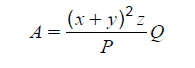x and y are both increased by 50 %

z is decreased by 20 %

P is doubled and Q remains the same.

4. What is the resulting percentage change in A?
0
0

## Explanation

Post Comment

The three statements below are about exhaling.

1. The ribs swing down and inwards during exhaling.

2. The diaphragm muscles contract during exhaling.

3. The pressure in the lungs increases during exhaling.

5. Which statements are correct?
0
0

## Explanation

Post Comment

The oscillation period, t, for a spiral spring (weight w, radius R made from wire length l, radius r with a modulus of rigidity n) with a weight W hanging from it is given by: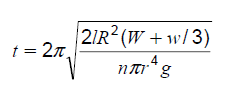If such a spring is used to measure the weight, W, of an object we need to rearrange the equation so W is the subject.

6. Which of the following is the correct rearrangement of the equation?
0
0

## Explanation

Post Comment

A 100 % efficient transformer has 1500 turns on its primary coil. The input to the transformer is 250 V ac. The output current is 10 A and the output power is 0.5 kW.

7. What is the number of turns on the secondary coil?
0
0

## Explanation

Post Comment

An oxide of iron has the formula Fe3O4 and contains both Fe2+ and Fe3+ ions.

8. Which one of the following is the fraction of iron ions that are in the Fe²⁺ state?
0
0

## Explanation

Post Comment

Which row of the table shows the state of the heart valves when the heart pumps blood to the lungs?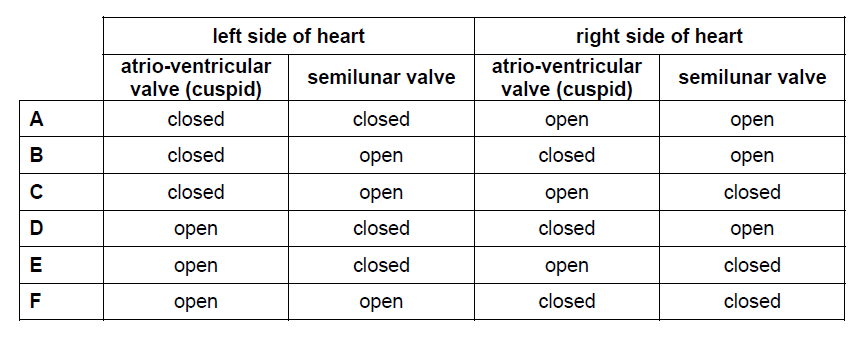9.
0
0

## Explanation

Post Comment

The reaction between lead(II) nitrate solution and potassium iodide solution is shown below:

Pb(NO3)2 (aq) + 2Kl (aq) → 2KNO3 (aq) + Pbl2 (s)

When the two solutions are mixed in a test tube the height of the lead(II) iodide precipitate formed can be measured.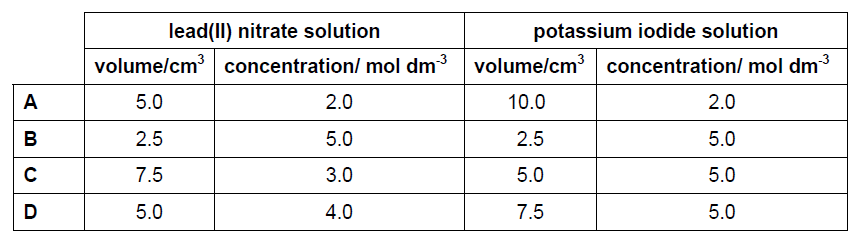10. Which combination of solutions would produce the greatest height of the precipitate?
0
0

## Explanation

Post Comment

Below are four statements about thermal (heat) energy.

1. A substance can lose heat energy without its temperature falling.

2. Heat energy can pass through a vacuum.

3. Steam at 100 oC has more heat energy than the same mass of boiling water at 100 oC

4. When a container of water is cooled near the top, a convection current is set up in the water.

11. Which statements are true?
0
0

## Explanation

Post Comment

The box and whisker plot shows some information about members of a health club using a walking machine. It shows the number of steps that a group of 30 members did in a period of 10 minutes.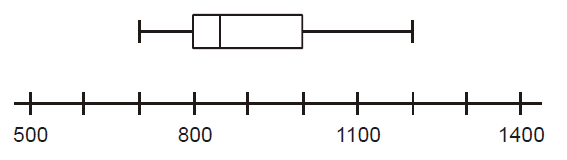Use this information to find the probability that when 3 of these 30 members are chosen at random, they will all have taken between 850 and 1000 steps.

12.
0
0

## Explanation

Post Comment

The diagram shows the bones and leg muscles of a person standing on their toes. The bones and muscles are acting as a lever system.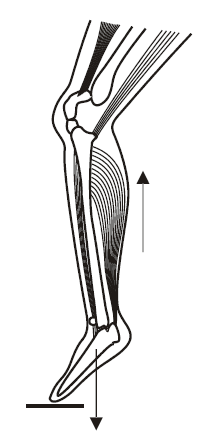13. Which of the following is the correct description of this lever system?
0
0

## Explanation

Post Comment

Which of the following reactions between a Group 1 element and a Group 7 element would be the most exothermic?

14.
0
0

## Explanation

Post Comment

Which diagram shows how a ray of white light is refracted by an air bubble in water, and gives the correct relative positions of the red and violet light formed by dispersion?

15.
0
0

## Explanation

Post Comment

The two inequalities given below define an area on a graph.

y x2 +3

x ≥ 1/ y

16. Which one of the following points lies within the area defined?
0
0

## Explanation

Post Comment

The below diagram shows a family tree of a condition known as nail patella syndrome (NPS).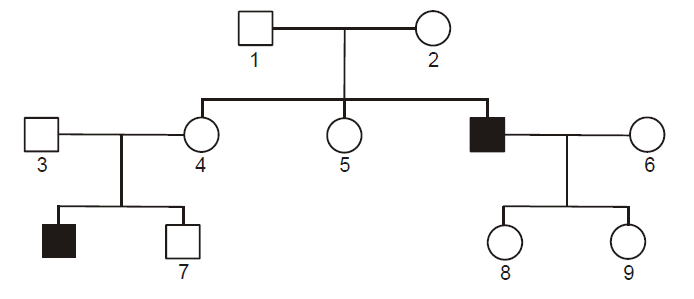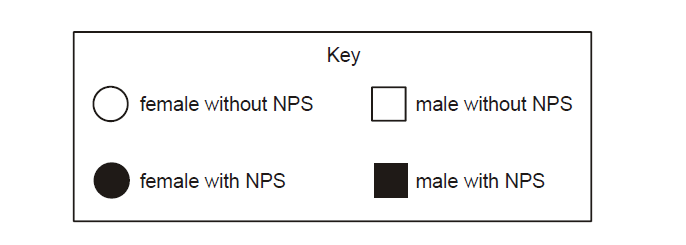17. Which of the following pairs of individuals must be heterozygous for NPS?
0
0

## Explanation

Post Comment

What volume of steam would be produced from an ice cube of mass 6.00 g if it were heated until it all evaporated?

(Ar values: H = 1; O = 16)

(Take molar volume at room temperature and pressure = 24 dm3)

18.
0
0

## Explanation

Post Comment

A heavy uniform bar is in equilibrium in the arrangement shown.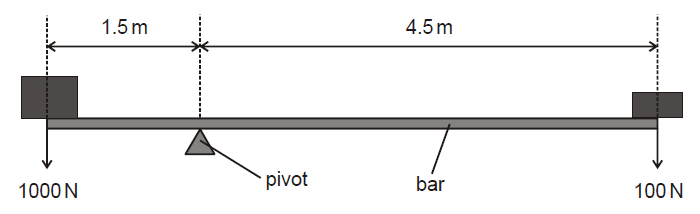19. What is the weight of the bar? (Give your answer to the nearest Newton.)
0
0

## Explanation

Post Comment

The longest side of a right angled triangle is 6 + √5 units. One of the shorter sides is 3 +2 √5 units.

20. What is the length of the third side?
0
0

## Explanation

Post Comment

A patient with leukaemia is being treated with chemotherapy and radiotherapy. The patient is found to have a low blood platelet count and a high percentage of the white blood cells are abnormal.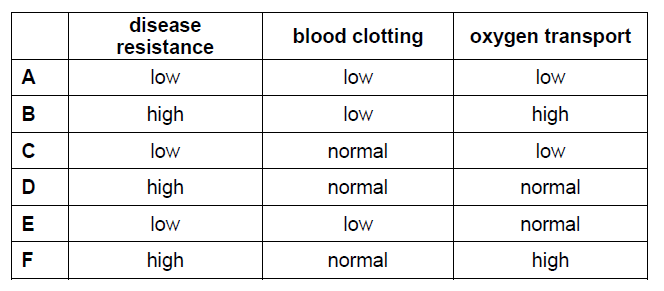21. Which row of the table shows this patient’s symptoms?
0
0

## Explanation

Post Comment

Some statements about ammonia are given below.

1. Its formula is NH3.

2. The pH of its solution in water is less than 7.

3. It has a molecular structure.

4. It turns damp blue litmus paper red.

5. It is a liquid at room temperature.

6. It is covalently bonded.

22. Which are correct statements about ammonia?
0
0

## Explanation

Post Comment

An artery has a length x mm and blood flows steadily through it at V ml per second.

23. If, on average, a given red blood cell takes T seconds to travel the length of the artery, what is the cross-sectional area of the artery in mm²?
0
3

## Explanation

Post Comment

The solid shown below consists of a cylinder topped by a hemisphere of the same radius.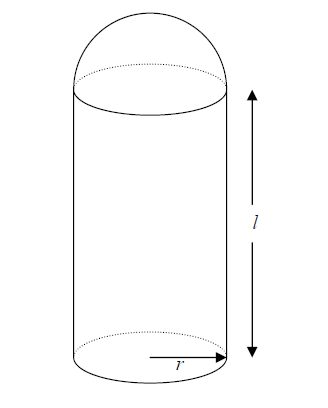24. Which one of the following correctly gives the volume of this solid?
0
0

## Explanation

Post Comment

A mixture of equal parts of hexane (bp 68 oC) and heptane (bp 98 oC) is distilled using a fractionating column.

The temperature of the liquid in the flask and the temperature at the top of the fractionating column are measured.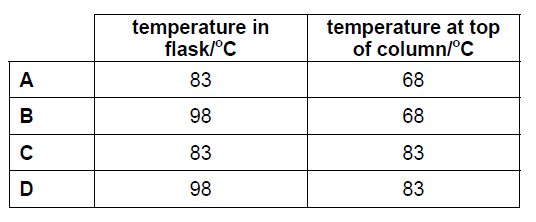25. Which one of the following shows the likely temperatures when the first drops of distillate are collected?
0
0

## Explanation

Post Comment

Which row of the table shows the roles of the female sex hormones in controlling the menstrual cycle?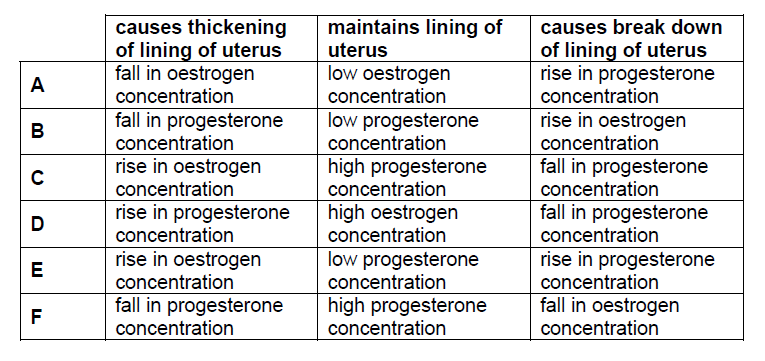26.
0
0

## Explanation

Post Comment

The position of cobalt in the periodic table is as follows: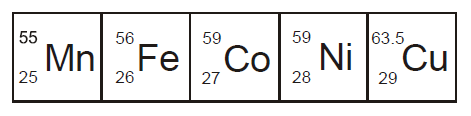Cobalt 60 is a radioactive isotope that decays with the emission of beta and gamma radiation. A beam of radiation from a cobalt 60 source is directed towards a malignant tumour in a patient’s abdomen.

1. The cobalt (27Co) is being converted into nickel (28Ni) as it decays.

2. The tumour is attacked by the gamma radiation.

3. The radiation attacks only the malignant cells.

27. Which of the following is/are correct when cobalt 60 is used in this way?
0
0

Post Comment

## Instructions

Below is a summary of your answers. You can review your questions in three (3) different ways.

The buttons in the lower right-hand corner correspond to these choices:

2. Review questions that are incomplete.
3. Review questions that are flagged for review. (Click the 'flag' icon to change the flag for review status.)

You may also click on a question number to link directly to its location in the exam.

## Instructions

This review section allows you to view the answers you made and see whether they were correct or not. Each question accessed from this screen has an 'Explain Answer' button in the top left hand side. By clicking on this you will obtain an explanation as to the correct answer.

At the bottom of this screen you can choose to 'Review All' answers, 'Review Incorrect' answers or 'Review Flagged' answers. Alternatively you can go to specific questions by opening up any of the sub-tests below.

x

## When should we call you?

It would be great to have a 15m chat to discuss a personalised plan and answer any questions

## What time works best for you? (UK Time)

Pick a time-slot that works best for you ?

Submit

Submit